A Source Book in Mathematics, 1200-1800

Contents:
Author: René Descartes  | Date: 1637

Show Summary
Mathematics

5 DESCARTES.

# The Equation of a Curve

I wish to know the genre1 of the curve EC [Fig. 1], which I imagine to be described by the intersection of the ruler GL and the rectilinear plane figure CNKL, whose side KN is produced indefinitely in the direction of C, and which,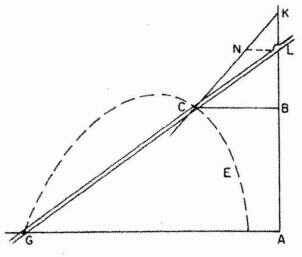Fig. 1

being moved in the same plane in such a way that its side KL always coincides with some part of the line BA (produced in both directions), imparts to the ruler GL a rotary motion about G (the ruler being so connected to the figure CNKL that it always passes through L).2 If I wish to find out to what genre this curve belongs, I choose a straight line, as AB, to which to refer all its points, and in AB I choose a point like A at which to begin the calculation. I say that I choose the one and the other, because we are free to choose them as we like, for while it is necessary to use care in the choice in order to make the equation as short and simple as possible, yet no matter what line I should take instead of AB the curve would always prove to be of the same genre, a fact easily demonstrated.

Then I take on the curve an arbitrary point, as C, at which I will suppose that the instrument to describe the curve is applied. Then I draw through C the line CB parallel to GA. Since CB and BA are unknown and indeterminate quantities, I shall call one of them y and the other x. But in order to find the relation between these quantities I consider also the known quantities which determine the description of the curve, as GA, which I shall call a; KL, which I shall call b; and NL, parallel to GA, which I shall call c. Then I say that as NL is to LK, or as c is to b, so CB, or y, is to BK, which is therefore equal to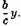Then BL is equal to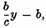and AL is equal to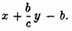Moreover, as CB is to LB, that is, as y is to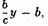so AG or a is to LA or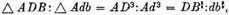Multiplying the second by the third, we get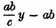equal to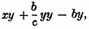which is obtained by multiplying the first by the last. Therefore, the required equation is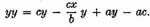From this equation we see that the curve EC belongs to the first genre, it being, in fact, a hyperbola.

If in the instrument used to describe the curve we substitute for the straight line CNK this hyperbola or some other curve of the first genre lying in the plane CNKL, the intersection of this curve with the ruler GL will describe, instead of the hyperbola EC, another curve, which will be of the second genre.

Thus, if CNK be a circle having its center at L, then we shall describe the first Conchoid of the Ancients,3 while if we use a parabola having KB as diameter we shall describe the curve which, as I have already said, is the first and simplest of the curves required in the problem of Pappus, that is, the one which furnishes the solution when five lines are given in position.4

1 Earlier in Book II, Descartes has defined the genre of a curve. In our terms: If an algebraic curve has degree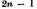its genre is n. This terminology may have boon inspired by the problem of Pappus. Newton (see Selection III.8) translates genre by genus.

2 The instrument thus consists of three parts: (1) a ruler AK of indefinite length, fixed in the plane; (2) a ruler GL, also of indefinite length, passing through a pivot G in this plane (but not on AK); and (3) a triangle LNK, KN indefinitely extended toward KC, to which the ruler GL is connected at L so as to make the triangle slide with its side KL along AB.

3 Pappus mentions four types of conchoid (shell curves); the first is the one we still call a conchoid, in polar coordinates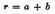sec θ. It is a curve of the third degree, therefore of the second genre of Descartes.

4 This is also a curve of the second genre.

Contents:

### Related Resources

René Descartes

Title: A Source Book in Mathematics, 1200-1800

Select an option:

## Email Options

Title: A Source Book in Mathematics, 1200-1800

Select an option: